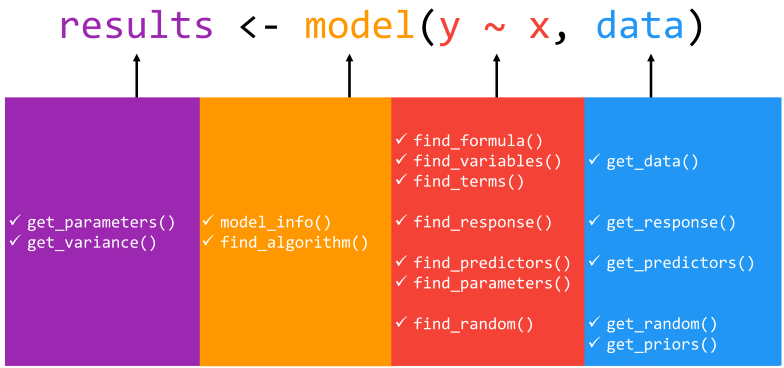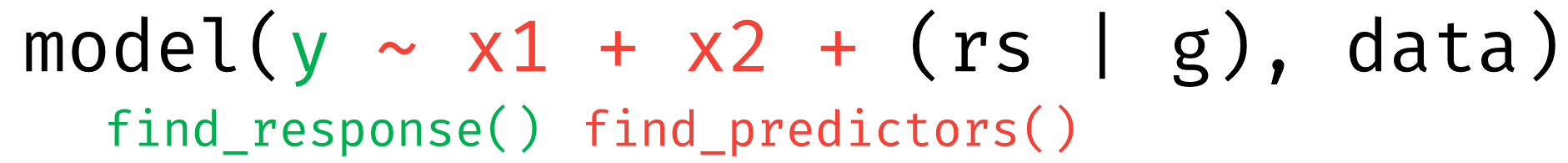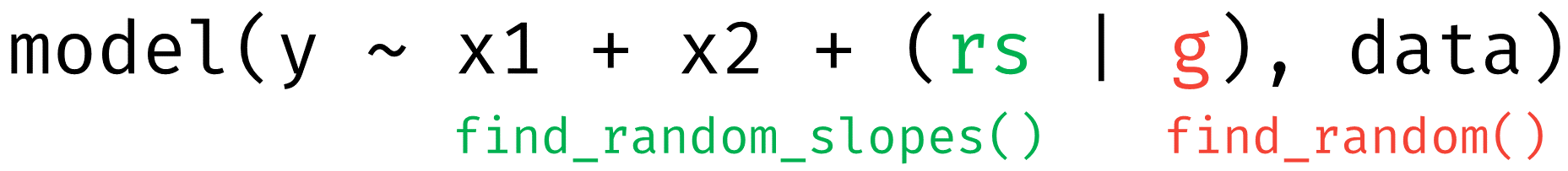# Getting Started with Accessing Model Information

When fitting any statistical model, there are many useful pieces of information that are simultaneously calculated and stored beyond coefficient estimates and general model fit statistics. Although there exist some generic functions to obtain model information and data, many package-specific modeling functions do not provide such methods to allow users to access such valuable information.

{insight} is an R-package that fills this important gap by providing a suite of functions to support almost any model. The goal of {insight}, then, is to provide tools to provide easy, intuitive, and consistent access to information contained in model objects. These tools aid applied research in virtually any field who fit, diagnose, and present statistical models by streamlining access to every aspect of many model objects via consistent syntax and output.

Built with non-programmers in mind, {insight} offers a broad toolbox for making model and data information easily accessible. While {insight} offers many useful functions for working with and understanding model objects (discussed below), we suggest users start with model_info(), as this function provides a clean and consistent overview of model objects (e.g., functional form of the model, the model family, link function, number of observations, variables included in the specification, etc.). With a clear understanding of the model introduced, users are able to adapt other functions for more nuanced exploration of and interaction with virtually any model object.

## Overview of Core Functions

A statistical model is an object describing the relationship between variables. Although there are a lot of different types of models, each with their specificities, most of them also share some common components. The goal of {insight} is to help you retrieve these components.

The get_* prefix extracts values (or data) associated with model-specific objects (e.g., parameters or variables), while the find_* prefix lists model-specific objects (e.g., priors or predictors). These are powerful families of functions allowing for great flexibility in use, whether at a high, descriptive level (find_*) or narrower level of statistical inspection and reporting (get_*). We point users to the package documentation or the complementary package website, https://easystats.github.io/insight/, for a detailed list of the arguments associated with each function as well as the returned values from each function.## Definition of Model Components

The functions from {insight} address different components of a model. In an effort to avoid confusion about specific “targets” of each function, in this section we provide a short explanation of {insight}’s definitions of regression model components.

### Data

The dataset used to fit the model.

### Parameters

Values estimated or learned from data that capture the relationship between variables. In regression models, these are usually referred to as coefficients.

### Response and Predictors

• response: the outcome or response variable (dependent variable) of a regression model.
• predictor: independent variables of (the fixed part of) a regression model. For mixed models, variables that are only in the random effects part (i.e. grouping factors) of the model are not returned as predictors by default. However, these can be included using additional arguments in the function call, treating predictors are “unique”. As such, if a variable appears as a fixed effect and a random slope, it is treated as one (the same) predictor.### Variables

Any unique variable names that appear in a regression model, e.g., response variable, predictors or random effects. A “variable” only relates to the unique occurrence of a term, or the term name. For instance, the expression x + poly(x, 2) has only the variable x.### Terms

Terms themselves consist of variable and factor names separated by operators, or involve arithmetic expressions. For instance, the expression x + poly(x, 2) has one variable x, but two terms x and poly(x, 2).### Random Effects

• random slopes: variables that are specified as random slopes in a mixed effects model.
• random or grouping factors: variables that are specified as grouping variables in a mixed effects model.## Examples

Aren’t the predictors, terms, and parameters the same thing?

In some cases, yes. But not in all cases, and sometimes it is useful to have the “bare” variable names (terms), but sometimes it is also useful to have the information about a possible transformation of variables. That is the main reason for having functions that cover similar aspects of a model object (like find_terms() and find_predictors() or find_variables()).

Here are some examples that demonstrate the differences of each function:

library(insight)
library(lme4)
data(sleepstudy)
sleepstudy$mygrp <- sample(1:5, size = 180, replace = TRUE) sleepstudy$mysubgrp <- NA
sleepstudy$Weeks <- sleepstudy$Days / 7
sleepstudy$cat <- as.factor(sample(letters[1:4], nrow(sleepstudy), replace = TRUE)) for (i in 1:5) { filter_group <- sleepstudy$mygrp == i
sleepstudy$mysubgrp[filter_group] <- sample(1:30, size = sum(filter_group), replace = TRUE) } model <- suppressWarnings(lmer( Reaction ~ Days + I(Days^2) + log1p(Weeks) + cat + (1 | mygrp / mysubgrp) + (1 + Days | Subject), data = sleepstudy )) # find the response variable find_response(model) #>  "Reaction" # find all predictors, fixed part by default find_predictors(model) #>$conditional
#>  "Days"  "Weeks" "cat"

# find random effects, grouping factors only
find_random(model)
#> $random #>  "mysubgrp:mygrp" "mygrp" "Subject" # find random slopes find_random_slopes(model) #>$random
#>  "Days"

# find all predictors, including random effects
find_predictors(model, effects = "all", component = "all")
#> $conditional #>  "Days" "Weeks" "cat" #> #>$random
#>  "mysubgrp" "mygrp"    "Subject"

# find all terms, including response and random effects
# this is essentially the same as the previous example plus response
find_terms(model)
#> $response #>  "Reaction" #> #>$conditional
#>  "Days"         "I(Days^2)"    "log1p(Weeks)" "cat"
#>
#> $random #>  "mysubgrp" "mygrp" "Days" "Subject" # find all variables, i.e. also quadratic or log-transformed predictors find_variables(model) #>$response
#>  "Reaction"
#>
#> $conditional #>  "Days" "Weeks" "cat" #> #>$random
#>  "mysubgrp" "mygrp"    "Subject"

Finally, there is find_parameters(). Parameters are also known as coefficients, and find_parameters() does exactly that: returns the model coefficients.

# find model parameters, i.e. coefficients
find_parameters(model)
#> $conditional #>  "(Intercept)" "Days" "I(Days^2)" "log1p(Weeks)" "catb" #>  "catc" "catd" #> #>$random
#> $random$mysubgrp:mygrp
#>  "(Intercept)"
#>
#> $random$Subject
#>  "(Intercept)" "Days"
#>
#> $random$mygrp
#>  "(Intercept)"

## Examples of Use Cases in R

We now would like to provide examples of use cases of the {insight} package. These examples probably do not cover typical real-world problems, but serve as illustration of the core idea of this package: The unified interface to access model information. {insight} should help both users and package developers in order to reduce the hassle with the many exceptions from various modelling packages when accessing model information.

### Making Predictions at Specific Values of a Term of Interest

Say, the goal is to make predictions for a certain term, holding remaining co-variates constant. This is achieved by calling predict() and feeding the newdata-argument with the values of the term of interest as well as the “constant” values for remaining co-variates. The functions get_data() and find_predictors() are used to get this information, which then can be used in the call to predict().

In this example, we fit a simple linear model, but it could be replaced by (m)any other models, so this approach is “universal” and applies to many different model objects.

library(insight)
m <- lm(
Sepal.Length ~ Species + Petal.Width + Sepal.Width,
data = iris
)

dat <- get_data(m)
pred <- find_predictors(m, flatten = TRUE)

l <- lapply(pred, function(x) {
if (is.numeric(dat[[x]]))
mean(dat[[x]])
else
unique(dat[[x]])
})

names(l) <- pred
l <- as.data.frame(l)

cbind(l, predictions = predict(m, newdata = l))
#>      Species Petal.Width Sepal.Width predictions
#> 1     setosa    1.199333    3.057333    5.101427
#> 2 versicolor    1.199333    3.057333    6.089557
#> 3  virginica    1.199333    3.057333    6.339015

### Printing Model Coefficients

The next example should emphasize the possibilities to generalize functions to many different model objects using {insight}. The aim is simply to print coefficients in a complete, human readable sentence.

The first approach uses the functions that are available for some, but obviously not for all models, to access the information about model coefficients.

print_params <- function(model){
paste0(
"My parameters are ",
paste0(row.names(summary(model)\$coefficients),  collapse = ", "),
", thank you for your attention!"
)
}

m1 <- lm(Sepal.Length ~ Petal.Width, data = iris)
print_params(m1)
#>  "My parameters are (Intercept), Petal.Width, thank you for your attention!"

# obviously, something is missing in the output
m2 <- mgcv::gam(Sepal.Length ~ Petal.Width + s(Petal.Length), data = iris)
print_params(m2)
#>  "My parameters are , thank you for your attention!"

As we can see, the function fails for gam-models. As the access to models depends on the type of the model in the R ecosystem, we would need to create specific functions for all models types. With {insight}, users can write a function without having to worry about the model type.

print_params <- function(model){
paste0(
"My parameters are ",
paste0(insight::find_parameters(model, flatten = TRUE),  collapse = ", "),
", thank you for your attention!"
)
}

m1 <- lm(Sepal.Length ~ Petal.Width, data = iris)
print_params(m1)
#>  "My parameters are (Intercept), Petal.Width, thank you for your attention!"

m2 <- mgcv::gam(Sepal.Length ~ Petal.Width + s(Petal.Length), data = iris)
print_params(m2)
#>  "My parameters are (Intercept), Petal.Width, s(Petal.Length), thank you for your attention!"

## Examples of Use Cases in R packages

{insight} is already used by different packages to solve problems that typically occur when the users’ inputs are different model objects of varying complexity.

For example, {ggeffects}, a package that computes and visualizes marginal effects of regression models, requires extraction of the data (get_data()) that was used to fit the models, and also the retrieval all model predictors (find_predictors()) to decide which covariates are held constant when computing marginal effects. All of this information is required in order to create a data frame for predict(newdata=<data frame>). Furthermore, the models’ link-functions (link_function()) resp. link-inverse-functions (link_inverse()) are required to obtain predictors at the model’s response scale.

The {sjPlot}-package creates plots or summary tables from regression models, and uses {insight}-functions to get model-information (model_info() or find_response()), which is used to build the components of the final plot or table. This information helps, for example, in labeling table columns by providing information on the effect type (odds ratio, incidence rate ratio, etc.) or the different model components, which split plots and tables into the “conditional” and “zero-inflated” parts of a model, in the cases of models with zero-inflation.

{bayestestR} mainly relies on get_priors() and get_parameters() to retrieve the necessary information to compute various indices or statistics of Bayesian models (like HDI, Credible Interval, MCSE, effective sample size, Bayes factors, etc.). The advantage of get_parameters() in this context is that regardless of the number of parameters the posterior distribution has, the necessary data can be easily accessed from the model objects. There is no need to write original, complicated code or regular expressions.

A last example is the {performance}-package, which provides functions for computing measures to assess model quality. Many of these indices (e.g. check for overdispersion or zero-inflation, predictive accuracy, logloss, RMSE, etc.) require the number of observations (n_obs()) or the data from the response-variable (get_response()). Again, in this context, functions from {insight} are helpful, because they offer a unified access to this information.